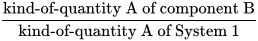Kind-of-quantity of dimension one - Dynamic NPU Manual
Select Page

# Content (cont.)

Synonym Dimensionless kind-of-quantity Quantity for which all the exponents of the factors corresponding to the base quantities in its quantity dimension are zero The measurement units and values of quantities of dimension one are numbers, but such quantities convey more information than a number. Some quantities of dimension one are defined as kind-of-quantity divided by the same kind-of-quantity The mathematical equation is:Examples are: mass fraction, substance ratio Other kinds-of-quantity of dimension one that may not be defined as above is Numbers of entities. International Vocabulary of Metrology – Basic and General Concepts and Associated Terms (VIM 3rd edition)JCGM 200:2012 https://jcgm.bipm.org/vim/en/1.8.html Ferard G, Dybkaer R, Fuentes-Arderiu X. Compendium of Terminology and Nomenclature of Properties in Clinical Laboratory Sciences : Recommendations 2016. 1 ed: Royal Society of Chemistry; 2016. 182 p. doi:10.1039/9781782622451.